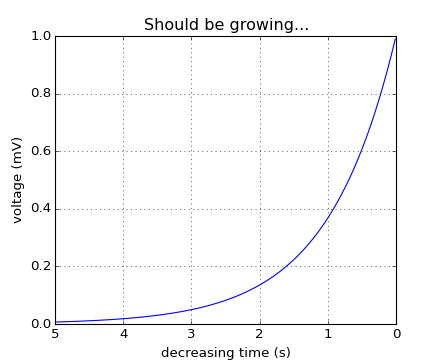### We're updating the default styles for Matplotlib 2.0

Learn what to expect in the new updates#### Previous topic

pylab_examples example code: interp_demo.py

#### Next topic

pylab_examples example code: layer_images.py

# pylab_examples example code: invert_axes.py¶```#!/usr/bin/env python
"""

You can use decreasing axes by flipping the normal order of the axis
limits

"""
from pylab import *

t = arange(0.01, 5.0, 0.01)
s = exp(-t)
plot(t, s)

xlim(5,0)  # decreasing time

xlabel('decreasing time (s)')
ylabel('voltage (mV)')
title('Should be growing...')
grid(True)

show()
```

Keywords: python, matplotlib, pylab, example, codex (see Search examples)